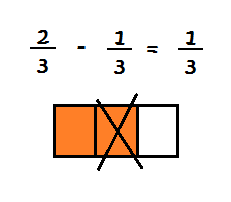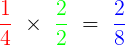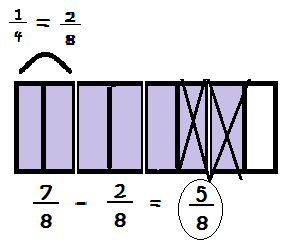# Rules for Subtracting Fractions

Instructor: Emily Hume

Emily is a Reading Specialist and Literacy coach in a public elementary school with a Master's Degree in Elementary Education.

Even though you may not realize it, fractions are all around you, so knowing how to work with them is an important skill. In this lesson, you will learn how to subtract fractions with like denominators as well as those with different denominators.

## Fraction Subtraction Action

You are sharing an apple with a friend. The apple is cut into three equal slices, or fractions, of the whole apple. Your friend quickly gobbles down a slice! Do you get your fair share of the apple, or did your friend eat too much? What fraction of the apple is left? You must subtract to find out.

However, like with so many things in life and in math, there are rules that must be followed in order to successfully engage in some fraction subtraction action. So, let's take a look!

## Rules for Subtracting Fractions

Rule 1: The fractions must have a common denominator.

What this means is that the denominators, or the bottom numbers, of the fractions, must be the same, what we call common denominators. If they are not the same, then we will find the common denominator and make them the same.

Rule 2: Once you have a common denominator, it does not change.

In other words, when you are subtracting your fractions, you are only subtracting the numerators, or the numbers on top.

Rule 3: Simplify the answer, if necessary.

If your answer has a numerator and a denominator that share a common factor, use that factor to reduce the fraction.

#### Example with Like Denominators

Here's an example of a fraction subtraction problem that already has common denominators:Since the denominators are the same, the only thing you have to do is subtract the top numbers! The denominator stays the same. So for this problem, 2 - 1 = 1, then the 3 stays the same so

2/3 - 1/3 = 1/3

#### Example with Different Denominators

Let's look at this fraction subtraction problem with different denominators:

7/8 - 1/4 = ?

By using equivalent fractions, fractions that look different but actually represent the same number, we can find the common denominator and can turn 1/4 into 2/8, which now has the same denominator as 7/8.So, 1/4 = 2/8. Here's a picture!Now that you have found the equivalent fraction to 1/4 (2/8), you are ready to subtract!

7/8 - 2/8 = 5/8 because 7 - 2 = 5 and the denominator (8) stays the same.

#### Subtraction Example Needing Simplifying

In this example, let's combine everything we've learned:

1/2 - 1/6 = ?

To unlock this lesson you must be a Study.com Member.

### Register to view this lesson

Are you a student or a teacher?

#### See for yourself why 30 million people use Study.com

##### Become a Study.com member and start learning now.
Back
What teachers are saying about Study.com

### Earning College Credit

Did you know… We have over 200 college courses that prepare you to earn credit by exam that is accepted by over 1,500 colleges and universities. You can test out of the first two years of college and save thousands off your degree. Anyone can earn credit-by-exam regardless of age or education level.